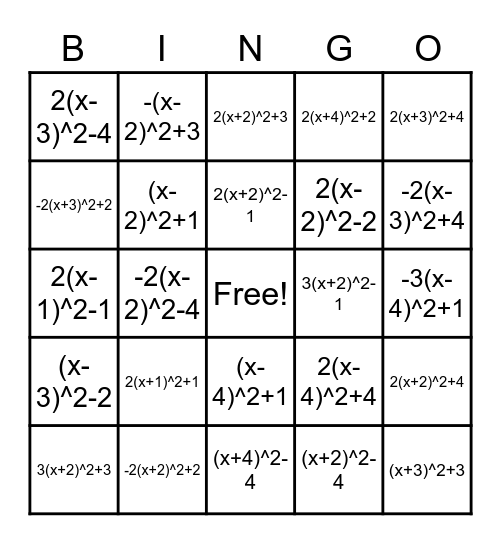# Vertex Form BingoThis bingo card has a free space and 29 words: 3(x+2)^2+3, -2(x-4)^2-3, -2(x-2)^2-4, (x-1)^2-2, 2(x-3)^2-4, 2(x+2)^2+3, (x-2)^2+1, 2(x+1)^2+1, 3(x+2)^2-1, -2(x+2)^2+2, -3(x-4)^2+1, (x+3)^2+3, (x+4)^2-4, (x+2)^2-4, -2(x-3)^2+4, 2(x-2)^2-3, (x-4)^2+1, (x-3)^2-2, 2(x+3)^2+4, 2(x-1)^2-1, 2(x-4)^2+4, (x-1)^2+1, 2(x-2)^2-2, -2(x+3)^2+2, 2(x+4)^2+2, -(x-2)^2+3, 2(x+2)^2+4, 2(x+2)^2-1 and 2(x+3)^2-1.

## Play Online